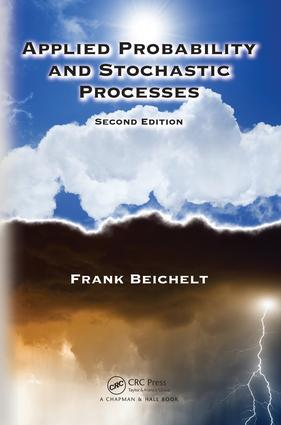# Applied Probability and Stochastic Processes

## 2nd Edition

Chapman and Hall/CRC

562 pages | 122 B/W Illus.

Hardback: 9781482257649
pub: 2016-05-03
SAVE ~\$19.39
\$96.95
\$77.56
x
eBook (VitalSource) : 9781315372334
pub: 2018-09-03
from \$48.48

FREE Standard Shipping!

### Description

Applied Probability and Stochastic Processes, Second Edition presents a self-contained introduction to elementary probability theory and stochastic processes with a special emphasis on their applications in science, engineering, finance, computer science, and operations research. It covers the theoretical foundations for modeling time-dependent random phenomena in these areas and illustrates applications through the analysis of numerous practical examples. The author draws on his 50 years of experience in the field to give your students a better understanding of probability theory and stochastic processes and enable them to use stochastic modeling in their work.

New to the Second Edition

• Completely rewritten part on probability theory—now more than double in size
• New sections on time series analysis, random walks, branching processes, and spectral analysis of stationary stochastic processes
• Comprehensive numerical discussions of examples, which replace the more theoretically challenging sections
• Additional examples, exercises, and figures

Presenting the material in a student-friendly, application-oriented manner, this non-measure theoretic text only assumes a mathematical maturity that applied science students acquire during their undergraduate studies in mathematics. Many exercises allow students to assess their understanding of the topics. In addition, the book occasionally describes connections between probabilistic concepts and corresponding statistical approaches to facilitate comprehension. Some important proofs and challenging examples and exercises are also included for more theoretically interested readers.

PROBABILITY THEORY

RANDOM EVENTS AND THEIR PROBABILITIES

RANDOM EXPERIMENTS

RANDOM EVENTS

PROBABILITY

CONDITIONAL PROBABILITY AND INDEPENDENCE OF RANDOM EVENTS

ONE-DIMENSIONAL RANDOM VARIABLES

MOTIVATION AND TERMINOLOGY

DISCRETE RANDOM VARIABLES

CONTINUOUS RANDOM VARIABLES

MIXTURES OF RANDOM VARIABLES

GENERATING FUNCTIONS

MULTIDIMENSIONAL RANDOM VARIABLES

TWO-DIMENSIONAL RANDOM VARIABLES

n-DIMENSIONAL RANDOM VARIABLES

FUNCTIONS OF RANDOM VARIABLES

FUNCTIONS OF ONE RANDOM VARIABLE

FUNCTIONS OF SEVERAL RANDOM VARIABLES

SUMS OF RANDOM VARIABLES

INEQUALITIES AND LIMIT THEOREMS

INEQUALITIES

LIMIT THEOREMS

STOCHASTIC PROCESSES

BASICS OF STOCHASTIC PROCESSES

MOTIVATION AND TERMINOLOGY

CHARACTERISTICS AND EXAMPLES

CLASSIFICATION OF STOCHASTIC PROCESSES

TIME SERIES IN DISCRETE TIME

RANDOM POINT PROCESSES

BASIC CONCEPTS

POISSON PROCESSES

RENEWAL PROCESSES

DISCRETE-TIME MARKOV CHAINS

FOUNDATIONS AND EXAMPLES

CLASSIFICATION OF STATES

LIMIT THEOREMS AND STATIONARY DISTRIBUTION

BIRTH AND DEATH PROCESSES

DISCRETE-TIME BRANCHING PROCESSES

CONTINUOUS-TIME MARKOV CHAINS

BASIC CONCEPTS AND EXAMPLES

TRANSITION PROBABILITIES AND RATES

STATIONARY STATE PROBABILITIES

SOJOURN TIMES IN PROCESS STATES

CONSTRUCTION OF MARKOV SYSTEMS

BIRTH AND DEATH PROCESSES

APPLICATIONS TO QUEUEING MODELS

SEMI-MARKOV CHAINS

MARTINGALES

DISCRETE-TIME MARTINGALES

CONTINUOUS-TIME MARTINGALES

BROWNIAN MOTION

INTRODUCTION

PROPERTIES OF THE BROWNIAN MOTION

MULTIDIMENSIONAL AND CONDITIONAL DISTRIBUTIONS

FIRST PASSAGE TIMES

TRANSFORMATIONS OF THE BROWNIAN MOTION

SPECTRAL ANALYSIS OF STATIONARY PROCESSES

FOUNDATIONS

PROCESSES WITH DISCRETE SPECTRUM

PROCESSES WITH CONTINUOUS SPECTRUM

REFERENCES

INDEX

Exercises appear at the end of each chapter.